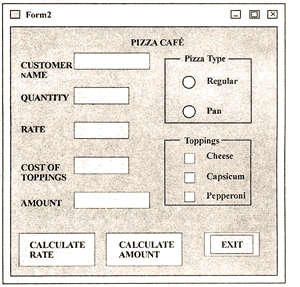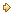Visitors Online: 77 | Saturday 24th August 2019

CBSE Guess > Papers > Question Papers > Class XII > 2006 > Informatics Practices > Delhi Set - I

Informatics Practices — 2006 (Set I — Delhi)

SECTION - A

Q. 1. Answer the following questions:

1. Explain the term Metadata using suitable example. 2
2. What is a Financial Accounting system? Name any two entities or tables that could be a part of this system. 2
3. What is feasibility study with reference to SDLC? Explain. 2
4. Explain the concept of object-oriented modeling. 2
5. Define Data Warehousing using suitable example. 2

Q. 2. Answer the following questions:

1. What do you understand by the term Event Driven Programming? Explain. 2
2. Write the names of any two properties and any two methods, which are common to the checkbox object and option button object. 2
3. Differentiate between the DriveListBox and DirListBox. Also name the most important property of each of these controls, which needs to be set by the user. 2
4. What is the purpose of the Common Dialog control? List any two methods of the Common Dialog Control. 2
5. What do you understand by the term data-bound controls? Name any two data-bound controls that can be used on a form. 2

Q. 3. Answer the following questions:

1. What do you understand by the term Equi - Join? Give an example. 2
2. Explain the difference between the SUBSTR and INSTR function of SQL with the help of an example. 2
3. What are group functions of SQL? Name any two group functions. 2
4. Differentiate between the COMMIT and ROLLBACK commands of SQL. 2
5. Explain the usage of the IN OUT parameter of a PL/SQL procedure with the help of an example. 2

SECTION – B

Q. 4. Read the following case study and answer the questions that follows:

The Pizza Cafe has computerized its billing. The following is the data entry screen used at their outlet. The outlet offers two different types of pizzas, regular and pan pizzas. The price of a regular pizza is Rs. 90 and that of a pan pizza Rs. 110. The user can choose to have three different types of extra toppings if he wants. Each extra toppings costs Rs. 20.The list for the above form is as follows

Object Type Object Name Description
Form frmPizza The Main Form Object
Text Box xtName To enter name of the customer
txtQty To enter number of Pizzas
txtRate To display rate of one pizza depending on its type
txtTopping To display cost of toppings
txtAmount To display total amount
Option Buttons optRegular To specify regular pizza
optPan To specify pan pizza
Check Boxed chikCheese To specify cheese topping
Chkcap To specify capsicum topping
Chkpep To specify pepperoni topping
Command Buttons cmdRate To calculate rate of pizza
cmdAmount To calculate the total amount
cmdExit To close the application
1. Write the commands to disable the textboxes txtKate, txt’l’opping, txtAmount and cmdAmount. 2
2. Write the code for cmdRate to calculate the rate of the pizza and display it in txtRate depending on the type of pizza selected by the customer. It should also enable the cmdAmount button. 4
3. Write the code for cmdAmount to calculate the total amount and display it in txtAmount. The total amount is calculated by first finding the cost of extra toppings selected by the customer. Remember that each extra topping costs Rs. 20 therefore if the user selects 2 toppings the cost of the toppings will be Rs. 40. Then add it to the rate and multiply the resultant amount by the quantity ordered. 4

Q. 5. Answer the following questions:

1. Find the errors from the following code segment and rewrite the corrected code underlining the correction made. 2
Dim num=10, las Integer
I = I
Do While 1 < 5
1 = 1 + 2
If num > 15
num = 0
Else
num = num — 3
Endlf
End While
2. Give output of the following statements: 2
(a) INSTR (LTRIM (“INTERNATIONAL”), “na”)
(b) INT (4 - 7 * 3/2 + 5)
3. Change the following code using FOR loop without effecting the output 2
Dim count, and
ans = 1
count = 20
DO
ans = ans * count
count = count -2
LOOP UNTIL count < = 10
Print ans
4. Write a Visual Basic procedure which takes a number as argument and dis plays whether it is prime or not. A prime number is one, which is not divisible by any number other than by 1 and by itself. 4

SECTION - C

Q. 6. Read the questions given below and answer accordingly:

1. Write the output produced by the following part of code in PL/SQL: 2
DECLARE
A NUMBER: = 10;
B NUMBER: = 2;
BEGIN
WHILE B < 12 LOOP
IF A > B THEN
DBMS_OUTPUT.PUT_LINE (TO_CHAR(A));
ELSE
DBMS_OUTPUT.PUT_LINE(TO_CHAR(B));
END IF;
A : = A — 4;
B : = B + 3;
END LOOP;
END;
2. Find the errors from the following PL/SQL code and rewrite the corrected code underlining the correction made. 2
CREATE ASWELLAS REPLACE TRIGGER DEPT_UP
AFTER UPDATE ON DEPT FOR_EVERY ROW
DECLARE
v_num NUMBER (3);
BEGIN
SELECT COUNT (*) INTO v_num FROM Emp WHERE Dept ‘1O1’;
IF v_num > 5
Raise_application_error (—20001, ‘Cannot exceed 5’);
END;
3. What do you understand by % ROWCOUNT with reference to cursors? What will happen if you use the %ROWCOUNT attribute of a cursor that is not open? 2
4. Write a PL/SQL procedure called FACTORIAL that takes an integer as parameter, finds its factorial and display it. (Factorial of a number is the product of all whole numbers less than and equal to that number. For example, factorial of 4 = 4 x 3 x 2 x 1 = 24). 4

Q. 7. Answer the questions base on the table CLUB given below:

Table : CLUB

 Column Name Data Type Size Constraint Description Member_No NUMBER 4 PRIMARY KEY Member Number Member_name VARCHAR2 30 NOT NULL Name of the member Address VARCHAR2 30 Address of the member Age NUMBER 3 > = 18 Age of the member Type VARCHAR2 10 Type of membership Fees NUMBER 6,2 Membership fees
1. Write the SQL command to create the table Club including the constraints. 2
2. Write the SQL command to display the details of the all the members whose type is “Permanent” and fees is more than Rs. 1000. 2
3. Write a Pl/SQL code to increase the fees by 5% for a member number accepted from the use if the type of the membership is “Temporary”. 3
4. Write Pl/SQL code using and explicit cursor to display the details of all the “permanent” members whose age is greater than 50. The code should also display the average membership fees of all such members. 3

 Informatics Practicess 2006 Question Papers Class XII Delhi Outside Delhi Compartment DelhiSet ISet ISet I

CBSE 2006 Question Papers Class XII